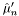#Boost C++ Libraries

...one of the most highly regarded and expertly designed C++ library projects in the world.

## Struct template covariance_impl

boost::accumulators::impl::covariance_impl — Covariance Estimator.

## Synopsis

```// In header: <boost/accumulators/statistics/covariance.hpp>

template<typename Sample, typename VariateType, typename VariateTag>
struct covariance_impl {
// types
typedef numeric::functional::average< Sample, std::size_t >::result_type             sample_type;
typedef numeric::functional::average< VariateType, std::size_t >::result_type        variate_type;
typedef numeric::functional::outer_product< sample_type, variate_type >::result_type result_type;

// construct/copy/destruct
template<typename Args> covariance_impl(Args const &);

// public member functions
template<typename Args> void operator()(Args const &) ;
result_type result(dont_care) const;
};```

## Description

An iterative Monte Carlo estimator for the covariance, whereis a sample andis a variate, is given by:

Equation 1.1.andbeing the means of the samples and variates.

### `covariance_impl` public construct/copy/destruct

1. `template<typename Args> covariance_impl(Args const & args);`

### `covariance_impl` public member functions

1. `template<typename Args> void operator()(Args const & args) ;`
2. `result_type result(dont_care) const;`